# Perimeter of Compound Shapes: Composite Rectangles

Perimeter of Compound Shapes: Composite Rectangles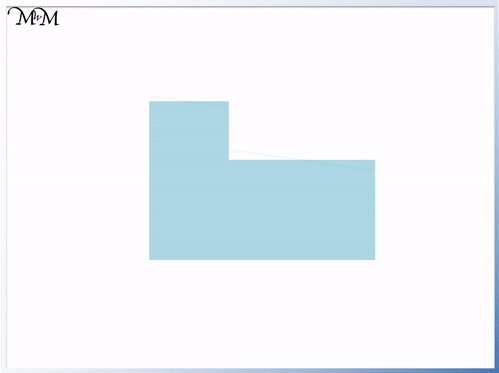• Perimeter is the total distance around the outside of a shape.
• The two green sides must be the same length in total as the one longer red side.
• The two green sides must be the same length in total as the one longer red side.
• We can use this fact to find missing sides before we add them to find the perimeter.• The missing side plus 6 cm must equal 9 cm. The missing vertical side is 3 cm.
• The missing side plus 4 cm must equal 12 cm. The missing horizontal side is 8 cm.
• We find the perimeter by adding the outside edges of our shape.
• 8 cm + 12 cm = 20 cm and 4 cm + 6 cm = 10 cm. We can add these four sides to make 30 cm
• We add the remaining two sides to 30 cm. 30 cm + 9 cm = 39 cm and then 39 cm + 3 cm = 42 cm.
• The perimeter of this compound shape is 42 cm.# Calculating the Perimeter of a Compound Shape made from Rectangles

A compound shape (or composite shape) is a more complex shape that is made up of two or more simple shapes.

We will be looking at compound shapes made specifically out of two combined rectangles.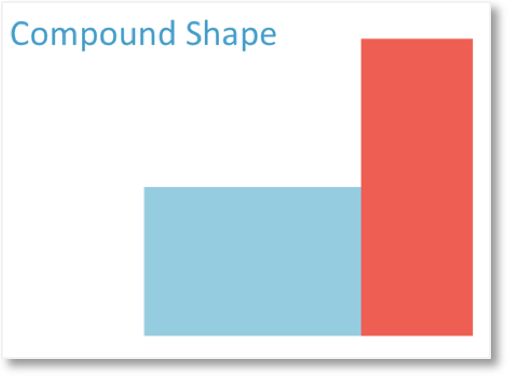We may be asked to find the perimeter of a compound shape like the one above.

For example:To find the perimeter, we simply add up the lengths of each outside edge.

It may be helpful to look out for number bonds when adding the sides.

For example, 7 + 3 = 10.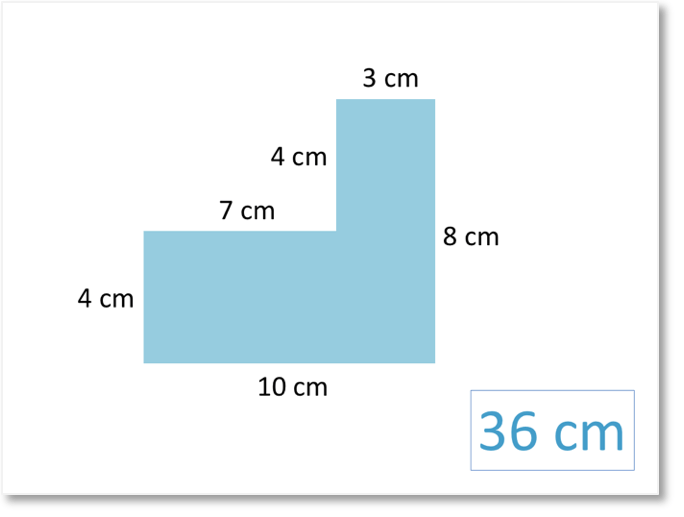The total of all of the outer sides is 36, so the perimeter is 36 cm.

In some perimeter questions, the lengths of some of the sides may be missing.

For example:Two of the side lengths of this rectangular composite shape are missing.

We can use the other side lengths to work out what these missing values are.We’ll begin with the missing

length.

To find its value, we will look at the other vertical lengths.Looking at the shape, the side that is 9 cm long is the same as both the missing side plus the side that is 6 cm long.

9 cm = ? cm + 6 cm

We can therefore find the missing length by subtracting 6 cm from 9 cm.

9 – 6 = 3

So, the missing side length is 3 cm.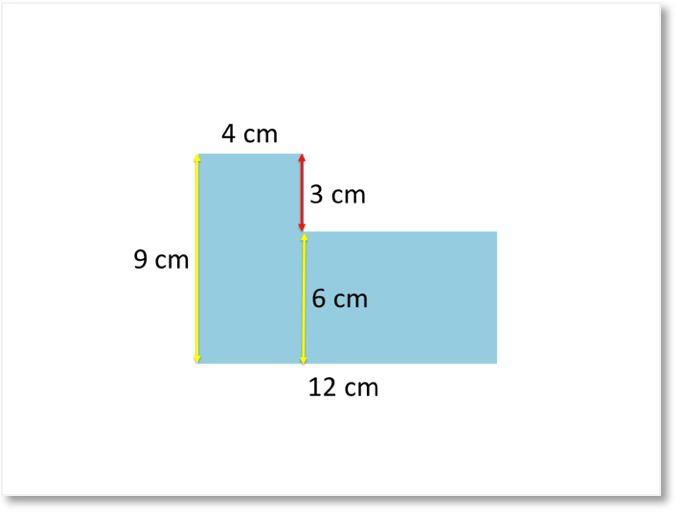Next, we’ll find the missing

length.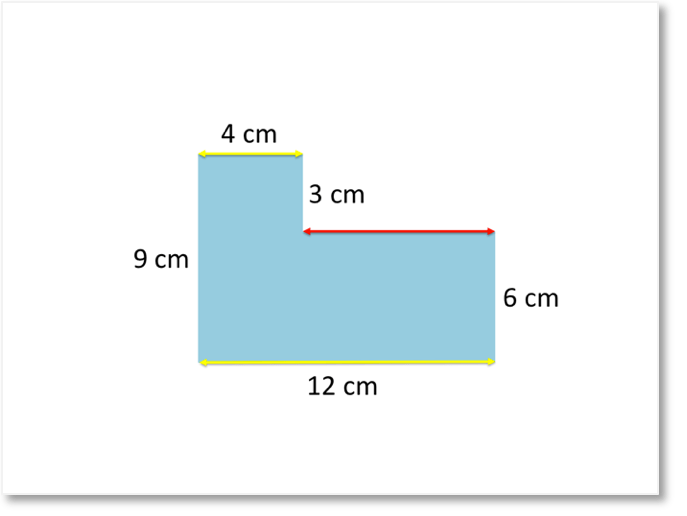To find the missing horizontal length, we will look at the values of the other horizontal lengths.Looking at the composite shape, the side that is 12 cm long is the same as both the missing side plus the side that is 4 cm long.

12 cm = 4 cm + ? cm

We can therefore find the missing length by subtracting 6 cm from 9 cm.

12 – 4 = 8

So, the missing length is 8 cm.Now that we know the lengths of all of the sides, we can find the perimeter of the composite shape.

Again, it may be helpful to look for number bonds to make the perimeter calculation easier.

For example, 12 + 8 = 20.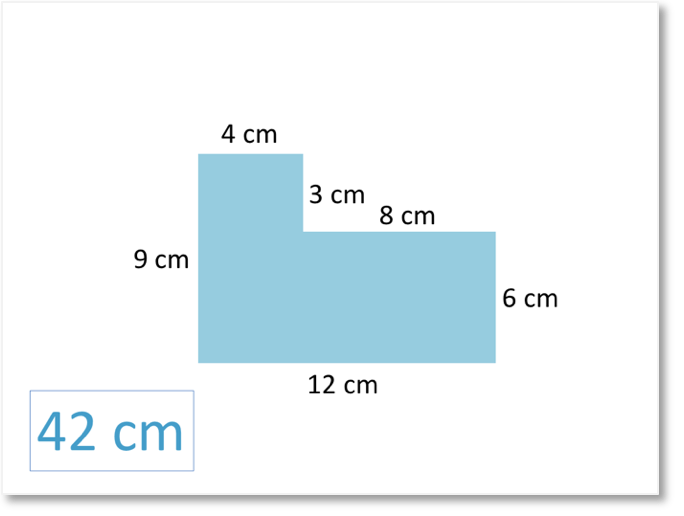The total is 42, so the perimeter of our composite shape is 42 cm.Now try our lesson on Calculating Area of Rectangles and Squares where we learn how to find the area of rectangles including squares.